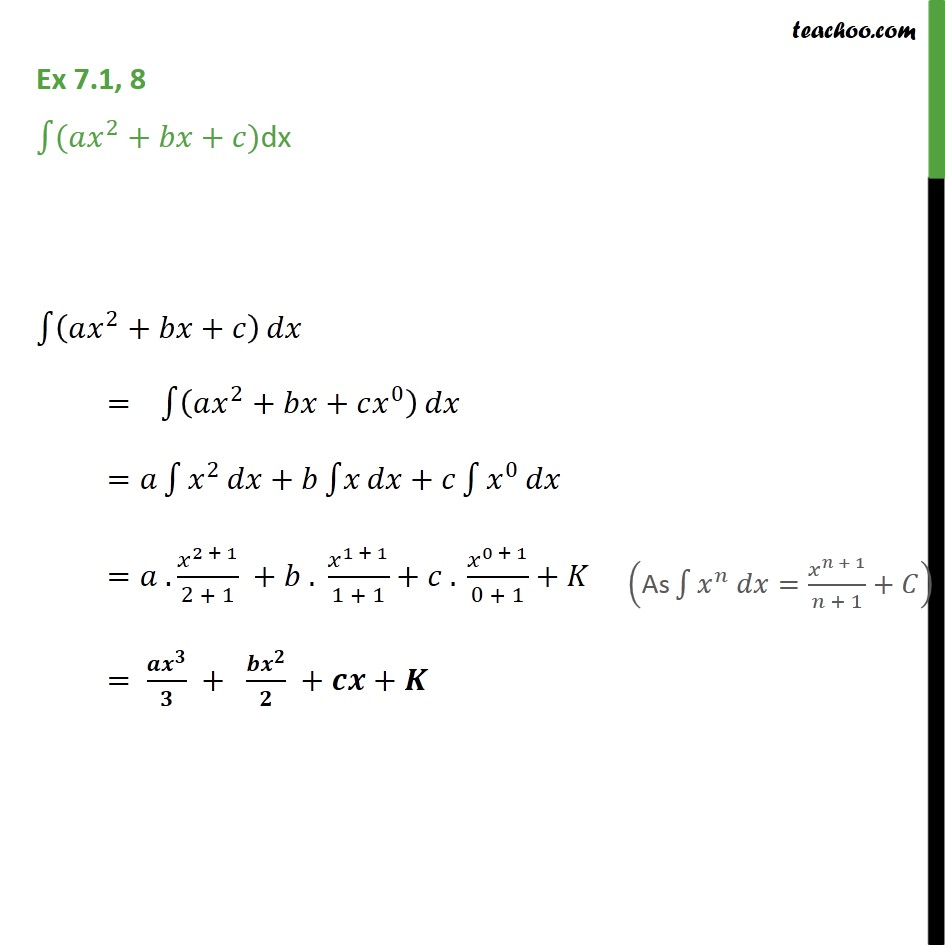1. Chapter 7 Class 12 Integrals (Term 2)
2. Serial order wise
3. Ex 7.1

Transcript

Ex 7.1, 8 ( 2 + + )dx 2 + + = 2 + + 0 = 2 + + 0 = . 2 + 1 2 + 1 + . 1 + 1 1 + 1 + . 0 + 1 0 + 1 + = + + +

Ex 7.1